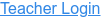• Probability
• Statistics
• Histograms

Description

Drop balls through a triangular grid of pegs and see them accumulate in containers. Switch to a histogram view and compare the distribution of balls to an ideal binomial distribution. Adjust the binary probability and develop your knowledge of statistics!

Sample Learning Goals

• Predict the bin where a single ball might fall
• Repeat trials of 100 balls and compare the outcomes
• Count the number of balls in a bin and relate that to the probability of falling in that bin
• Compare and interpret empirical and theoretical statistics
• Apply the plinko simulation as a model to other scenarios where there are weighted statistics
Version 1.1.3